Life Cycle
Life Cycle
State-of-the-Art Review

# Kaplan-Meier and Cox proportional hazards regression in survival analysis: statistical standard and guideline of Life Cycle Committee

1Department of Precision Medicine, Sungkyunkwan University School of Medicine, Suwon, Korea
*Correspondence: Seung Won Lee, E-mail: lsw2920@gmail.com

© Copyright 2023 Life Cycle. This is an Open-Access article distributed under the terms of the Creative Commons Attribution Non-Commercial License (http://creativecommons.org/licenses/by-nc/4.0/) which permits unrestricted non-commercial use, distribution, and reproduction in any medium, provided the original work is properly cited.

Received: Feb 12, 2023; Revised: Apr 19, 2023; Accepted: Apr 27, 2023

Published Online: May 17, 2023

## Abstract

In medical research, analyzing the time it takes for a phenomenon to occur is sometimes crucial. However, various factors can contribute to the length of survival or observation periods, and removing specific data can lead to bias results. In this paper, we discuss the Kaplan-Meier analysis and Cox proportional hazards regression model, which are the most frequently used methods in survival analysis. For the first step, we shall discuss the temporal concepts needed in survival analysis, such as cohort studies and then the basic statistical functions dealt with in survival analysis. After solidifying the concepts, methods of understanding and practical application of the Kaplan-Meier survival analysis is noted. After that, we will discuss the analysis methods for the Cox proportional hazards regression model, which includes multiple covariates. With the interpretation method of Cox proportional hazards regression result, we then discuss methods for checking the assumptions of the Cox proportional hazards regression, such as log minus log plots. Finally, we briefly explain the concept of time-dependent regression analysis. It is our aim that through this paper, readers can obtain an understanding on survival analysis and learn how to perform it.

Keywords: Cox proportional hazards regression; Kaplan-Meier; survival analysis

## 1. Introduction

The most significant difference between retrospective cohort studies and randomized controlled trials (RCTs) compared to cross-sectional studies lies in their temporal characteristics. In cohort studies, not only is a certain phenomenon (Y) important, but the analysis of the time it takes for the phenomenon to occur (time-to-event) is also crucial. However, when analyzing the time-to-event values, it is essential to consider that not all observations are complete data. The term “complete” used in this study refers to securing the same observation period for all patients without censoring. For example, there can be a situation where we designed a study to evaluate the effects of a specific drug on cancer patients’ survival. In this case, equally important as survival or death (Y = 0 or 1) is the survival time (time-to-event until death) for both group T (treatment group) and group C (control group). Thus, the dependent variable is a pair of outcome and survival time.

However, when comparing the survival time values of patients in group T and group C using the t-test of the average survival time variable, the following issue arises: “Should we exclude all data in cases where loss to follow-up occurred due to accidents, moving, tracking failure, research fund exhaustion, or death of observers?” Patients’ observation periods can be reduced for various reasons, which is defined as censoring. Since indiscriminately removing censored data can lead to bias issues, statistics that include this censored data must be used. Moreover, as some patients may have different starting points for the study, the initial observation starting point and end point for each patient can be very diverse as shown in the figure (Fig. 1). Survival analysis is a research method that targets both survival time and observation results in order to solve all these problems.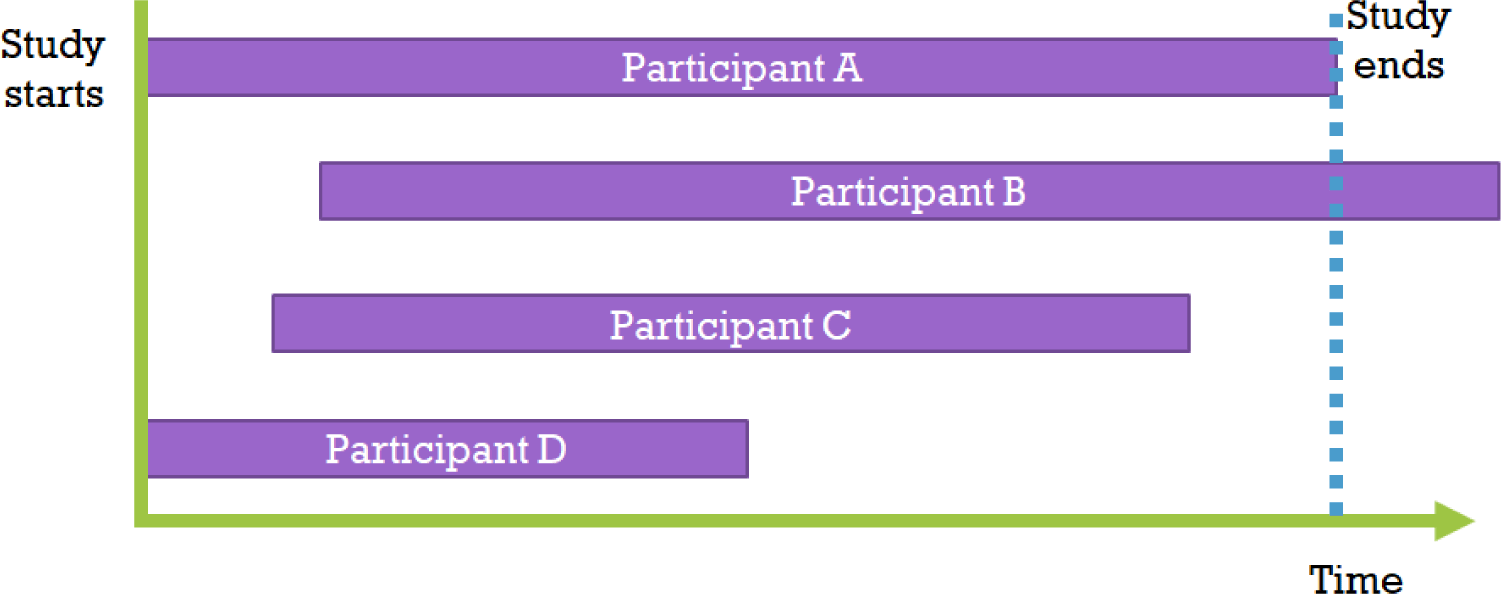Fig. 1. Variety of study participants

## 2. Main: survival analysis

2.1 Survival analysis related statistical functions

Survival analysis tools treat an individual’s survival time T as a random variable. That is, various survival analysis functions are defined and used for arbitrary values (survival time t) that the random variable T can take. These survival analysis functions include the following:

1. Survival function. S(t) = P(T > t) is a function representing the probability of being alive at a specific time point t, which means the probability of event time T being greater than t if the sample has not died on the research start date, S(0) = 1. As t in S(t) increases, the value of S(t) either remains the same or decreases (monotonically decreasing characteristic).

2. Lifetime distribution function. F(t) = 1–S(t), which is the probability that an event has occurred up to a specific time point t, opposite to the survival function. The lifetime distribution function F(t) is a type of cumulative function. The function f(t), which is the original form of this cumulative function, is the derivative of F(t) with respect to time, and is called the survival distribution density. f(t) can be interpreted as the death rate per unit time at the time point t.

3. Hazard function. h(t) = f(t)/S(t). This is the conditional probability that an event will occur immediately after surviving up to time t. The probability of an individual survivor who has survived up to day t and dying on day t is obtained by dividing the number of deaths occurring on day t, f(t), by the number of survivors remaining alive up to day t, S(t). Also, there is a cumulative hazard function H(t), which is the integral function of h(t).

2.2 Kaplan-Meier survival analysis

Kaplan-Meier survival analysis is a statistical technique for estimating the survival function. It corresponds to non-parametric statistics, which means that it does not assume parameters and calculates probabilities directly from the given data, regardless of the population’s distribution shape. In other words, it does not include normal distribution assumptions, allowing more general use of the data. According to the Kaplan-Meier estimator method, the data is first arranged in order from the shortest to the longest observation period, and then the starting points are all aligned to 0 (Fig. 2).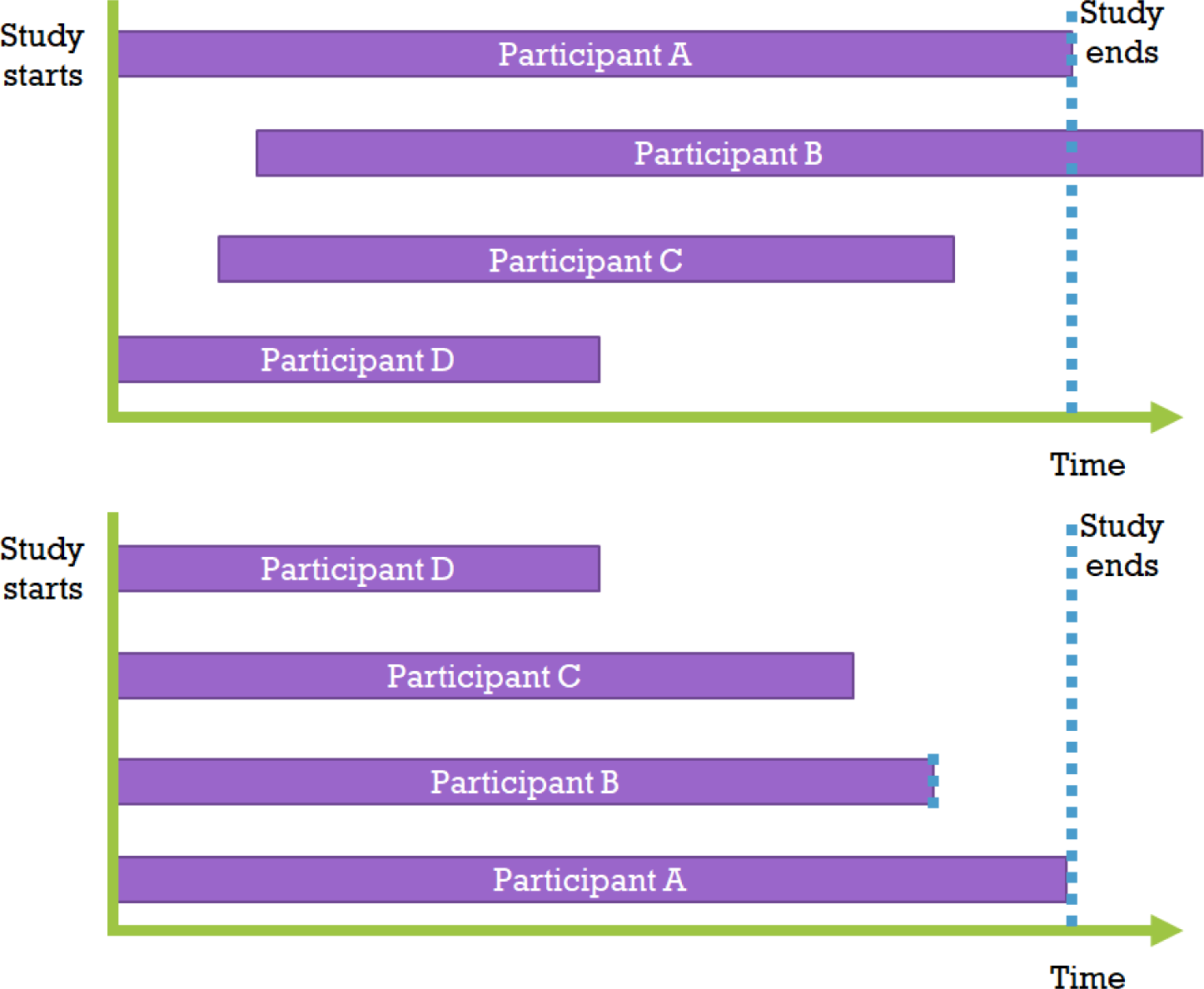Fig. 2. Arrange the data in order, unifying the observation points
2.3 Kaplan-Meier survival analysis and result interpretation using R

Examining the following example can help understand how to interpret the results of survival analysis in practice. The attached Cancer.csv file is Edmunson’s ovarian cancer research data (Table 1) . Applying Edmunson’s study, we examined whether patients who used a newly developed anticancer drug for ovarian cancer (treatment = 2) survived longer than those who used the existing anticancer drug (treatment = 1) using Kaplan-Meier analysis. The observation time (variable name: time) is the number of days from the start of treatment to the occurrence of death or the end of follow-up.

Table 1. Example data
ID Treatment (yes or no) Month Death Age, years Residual Condition
1 1 2 1 72.3315 2 1
2 1 3 1 74.4932 2 1
3 1 5 1 66.4658 2 2
4 2 14 0 53.3644 2 1
5 1 14 1 50.3397 2 1
6 1 14 0 56.4301 1 2
7 2 15 1 56.937 2 2
8 2 15 1 59.8548 2 2
9 1 15 0 64.1753 2 1
10 2 18 1 55.1781 1 2
11 1 21 1 56.7562 1 2
12 2 24 0 50.1096 1 1
13 2 25 0 59.6301 2 2
14 2 25 0 57.0521 2 1
15 1 26 0 39.2712 1 1
16 1 28 0 43.1233 1 2
17 1 34 0 38.8932 2 2
18 1 36 0 44.6 1 1
19 2 37 0 53.9068 1 1
20 2 40 0 44.2055 2 1
21 2 40 0 59.589 1 2
22 1 8 1 74.5041 2 2
23 1 11 1 43.137 2 1
24 2 11 1 63.2192 1 2
25 2 12 1 64.4247 2 1
26 2 12 0 58.3096 1 1

library(survival)

Surv(time, death)

f1 <- survfit(Surv(time, death) ~ treatment, data = cancer)

First, the load of the survival library in R and change of the research outcome pair, survival time and survival status, into a special variable (Surv). Then, the survival results (Surv) can be fitted to the Kaplan-Meier method according to the treatment group. The last line is the code that fit this into the Survfit function, which will obtain the resulting model f1.

In most cases, survival analysis compares the Kaplan-Meier survival curves of two groups. The comparison method used is the log-rank test, with the alternative hypothesis that the survival curves of the treatment and control groups are different. When comparing three or more groups, each are compared using the post-hoc test adjustment. The following is the code to visualize the results of survival analysis using the Survminer library in order to obtain confidence intervals and to obtain the P-value of the log-rank test.

Library(survminer)

ggsurvplot(f1, conf.int=TRUE, risk.table = TRUE, pval = TRUE)

When looking at the results of the code execution in terms of simple survival, it can be found that the new drug treatment group appears to have survived longer. However, the graph shows an overlapping of 95% confidence intervaks, and furthermore, the log-rank test outputs P-value=0.3. In conclusion, it is determined that the new drug did not significantly increase survival (Fig. 3).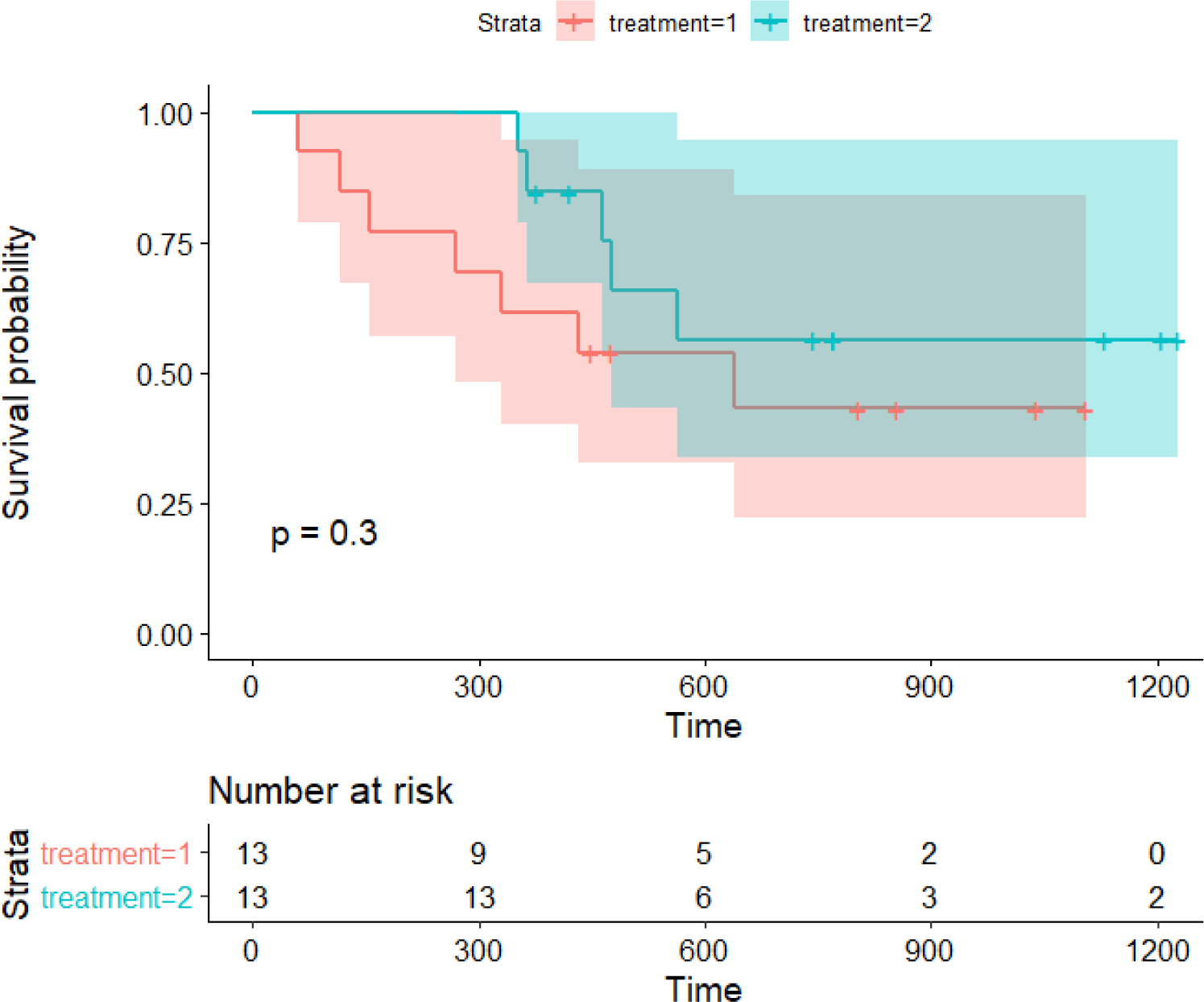Fig. 3. Kaplan-Meier analysis of Edmunson’s ovarian cancer research data

The fact that the proportional hazards assumption is a prerequisite for using the log-rank test described above must always be taken into consideration as it is the assumption that the hazard ratio remains constant throughout the study period. A constant hazard ratio means that the mortality rate of the treatment group/control group is always constant from day 1, day 2, ..., until the end of the study.

Kaplan-Meier survival analysis focuses only on the observation period and the occurrence of events. Therefore, other risk factors (such as gender and age) are not considered. Having no covariates in actual medical practice, not experimental studies, is rare. Nonetheless, in an RCT case, variables other than placebo and treatment drugs are randomly assigned and can be excluded from the model, so it is often used in such cases. However, in most studies where the match of other covariates cannot be assured, the Cox proportional hazards regression model, which will be discussed later, should be used.

2.4 Cox proportional hazards regression model

The basic Cox proportional hazards regression model assumes, like the Kaplan-Meier survival analysis, that the hazard ratio remains constant. The difference from it and the Kaplan-Meier survival analysis is that Cox proportional hazards regression models can analyze other variables that affect the occurrence of events. This is often the reason why the Cox proportional hazards model is used in most data studies.[10-13]

In the proportional hazards regression model, unlike the Kaplan-Meier analysis, an assumption about the original form of the survival function is needed. In the Cox proportional hazards regression model, this function is assumed to be an exponential function, such as s(t)=exp(–kt). Also, the hazard ratio must always be constant over time, which is called the proportional hazards assumption.

Under such assumptions, like in ordinary regression analysis, the hazard ratio of each covariate can be estimated and significant results can be obtained. In most cases, the hazard ratio can be interpreted in a similar way to the relative risk. The significance is evaluated based on whether the confidence interval includes 1 or not, and the value of the hazard ratio itself is given a quantitative meaning.

After analyzing the Cox proportional hazards regression model, survival functions and cumulative hazard functions are graphically represented as in the survival analysis. Similarly, it is common to display censored data, the number of survivors at each time point, etc. It is also often necessary to represent figures most commonly used for testing the proportional hazards assumption, such as the log minus log plot. The log minus log plot is a graph that performs log-log transformation on the survival function and outputs it for each value of the categorical variable; if there is an intersection in the graph, it can be determined that the proportional hazards assumption has been violated.

2.5 Cox time-dependent regression model for violated proportional hazards assumption

If an intersection is confirmed in the log minus log plot, the proportional hazards assumption is violated, meaning that the hazard ratio changes over time. In such cases, the time-dependent Cox regression can be performed to analyze changes in variables over time. In fact, many clinical variables strictly correspond to time-dependent variables. Vital sign values, blood test values, etc., are typical examples of time-dependent variables that change over time. Moreover, even if there is a constant value without time-dependency, a time-dependency could be hidden; for example, even if the same drug dosage is set daily, its effect may decrease as resistance develops.

When dealing with time-dependent variables, it may be appropriate to divide them based on the time-dependent cycle and assign them to each observation period. For example, in a study that checks for deaths on a daily basis and performs blood tests every week (every 7 days), data can be split at 7-day intervals and the method of using the blood test value variables for that week is possible.[11, 21]

## 3. Conclusion

Survival analysis has established itself as a very crucial research methodology in the medical field where observation time is important. Through survival analysis, such as the Kaplan-Meier analysis, the incidence of each group over time can be verified, and testing the differences between groups is possible. Furthermore, by using the Cox proportional hazards regression model, the hazard ratio of each group can be estimated quantitatively. As it is also possible when covariates are present, such methods are very useful for real world data research. However, testing the proportional hazards assumption, such as with the log minus log plot, is necessary in the progress. Finally, time-dependent Cox regression can be used for data with time-dependency using the time-dependent Cox regression.

Capsule Summary

This statistical standard and guideline of Life Cycle Committee summarizes the Kaplan-Meier analysis and Cox proportional hazards regression model, which are the most frequently used methods in survival analysis

## Patient and public involvement

No patients were directly involved in designing the research question or in conducting the research. No patients were asked for advice on interpretation or writing up the results. There are no plans to involve patients or the relevant patient community in dissemination at this moment.

## Transparency statement

The leading authors (Dr. SWL) are an honest, accurate, and transparent account of the study being reported.

None

## Author contribution

Dr SWL contributed to the preparation of this review. Life Cycle Committee (Dong Keon Yon [Seoul National University] and Youn Ho Shin [CHA University] approved the final version before submission.

## Funding

This work was supported by the National Research Foundation of Korea (NRF) grant funded by the Korea government (2021R1I1A2059735). The funders had no role in study design, data collection, data analysis, data interpretation, or writing of the report.

## Competing interests

The authors have no conflicts of interest to declare for this study.

## Provenance and peer review

Not commissioned; externally peer reviewed.

## References

2.

Kuitunen I, Ponkilainen VT, Uimonen MM, Eskelinen A, Reito A. Testing the proportional hazards assumption in cox regression and dealing with possible non-proportionality in total joint arthroplasty research: Methodological perspectives and review. BMC Musculoskelet Disord. 2021; 22((1)):489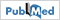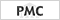4.

Woo A, Lee SW, Koh HY, Kim MA, Han MY, Yon DK. Incidence of cancer after asthma development: 2 independent population-based cohort studies. J Allergy Clin Immunol. 2021; 147((1)):135-435.

Lee SW. Methods for testing statistical differences between groups in medical research: statistical standard and guideline of Life Cycle Committee. Life Cycle. 2022; 2:e16.

Coemans M, Verbeke G, Döhler B, Süsal C, Naesens M. Bias by censoring for competing events in survival analysis. Bmj. 2022; 378e0713497.

Hernández-Herrera G, Moriña D, Navarro A. Left-censored recurrent event analysis in epidemiological studies: a proposal for when the number of previous episodes is unknown. BMC Med Res Methodol. 2022; 22((1)):208.

Edmonson JH, Fleming TR, Decker DG, Malkasian GD, Jorgensen EO, Jefferies JA, et al. Different chemotherapeutic sensitivities and host factors affecting prognosis in advanced ovarian carcinoma versus minimal residual disease. Cancer Treat Rep. 1979; 63((2)):241-79.

Goldenberg I, Kutyifa V, Klein HU, Cannom DS, Brown MW, Dan A, et al. Survival with cardiac-resynchronization therapy in mild heart failure. N Engl J Med. 2014; 370((18)):1694-70110.

Noh Y, Jeong HE, Choi A, Choi EY, Pasternak B, Nordeng H, et al. Prenatal and infant exposure to acid-suppressive medications and risk of allergic diseases in children. JAMA Pediatr. 2023; 177((3)):267-7712.

Yin Z, Zheng C, Fang Q, Gong X, Cao G, Li J, et al. Introduction of two-dose mumps-containing vaccine into routine immunization schedule in Quzhou, China, using cox-proportional hazard model. J Immunol Res. 2021; 2021:599041713.

Peng Y, Yu B, Kong DG, Zhao YY, Wang P, Pang BB, et al. Reinfection hazard of hand-foot-mouth disease in Wuhan, China, using Cox-proportional hazard model. Epidemiol Infect. 2018; 146((10)):1337-4214.

Rulli E, Ghilotti F, Biagioli E, Porcu L, Marabese M, D’Incalci M, et al. Assessment of proportional hazard assumption in aggregate data: a systematic review on statistical methodology in clinical trials using time-to-event endpoint. Br J Cancer. 2018; 119((12)):1456-6315.

du Prel JB, Hommel G, Röhrig B, Blettner M. Confidence interval or p-value?: part 4 of a series on evaluation of scientific publications. Dtsch Arztebl Int. 2009; 106((19)):335-917.

Hashemi R, Commenges D. Correction of the p-value after multiple tests in a Cox proportional hazard model. Lifetime Data Anal. 2002; 8((4)):335-4818.

Cheung CC, Vittinghoff E, Marcus GM, Gerstenfeld EP. Beware of the hazards: limitations of the proportional hazards assumption. Europace. 2021; 23((12)):204819.

Austin PC, Latouche A, Fine JP. A review of the use of time-varying covariates in the Fine-Gray subdistribution hazard competing risk regression model. Stat Med. 2020; 39((2)):103-1320.

Lee SW, Yang JM, Yoo IK, Moon SY, Ha EK, Yeniova A, et al. Proton pump inhibitors and the risk of severe COVID-19: a post-hoc analysis from the Korean nationwide cohort. Gut. 2021; 70((10)):2013-521.

Zadkarami MR. Applied shared log-normal frailty Cox-proportional hazard model to evaluating the effect of vitamin a on the rat passive avoidance memory. Pak J Biol Sci. 2008; 11((9)):1263-7